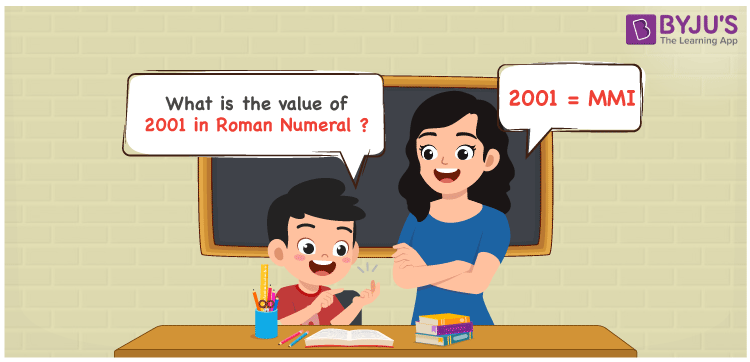# 2001 in Roman Numerals

2001 in Roman Numerals is MMI. Roman numerals is an additive and subtractive system where letters are used to show certain numbers in the number system. A different combination of symbols are used to indicate the roman numerals that include the English alphabet. Therefore, 2001 can be written as MMI in roman numerals.

 Number Roman Numeral 2001 MMI## How to Write 2001 in Roman Numerals?

In order to write 2001 in Roman Numerals, it has to be written in expanded form, i.e.

2001 = 2000 + 1

2001 = MM + I

2001 = MMI

## Video Lesson on Roman Numerals## Frequently Asked Questions on 2001 in Roman Numerals

Q1

### Write 2001 in Roman Numerals.

In Roman Numerals, 2001 is written as MMI.
Q2

### How to convert 2001 into Roman Numerals?

The conversion of 2001 into Roman Numerals includes breaking the number according to its place value.
Thousands = 2000 = MM
Ones = 1 = I
Number = 2000 + 1 = MM + I = MMI
Q3

### Why is 2001 in Roman Numerals Written as MMI?

We know that in roman numerals, we write 1 as I, and 1000 as M.
Therefore, 2001 in roman numerals is written as 2001 = 2000 + 1 = MM + I = MMI.